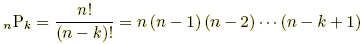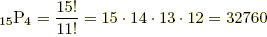# permutation formula

Permutation Formula

A formula for the number of possible permutations of k objects from a set of n. This is usually written nPk .

 Formula:Example: How many ways can 4 students from a group of 15 be lined up for a photograph? Answer: There are 15P4 possible permutations of 4 students from a group of 15.different lineups